# Master Division With Vedic Maths

###### ByRishi Raj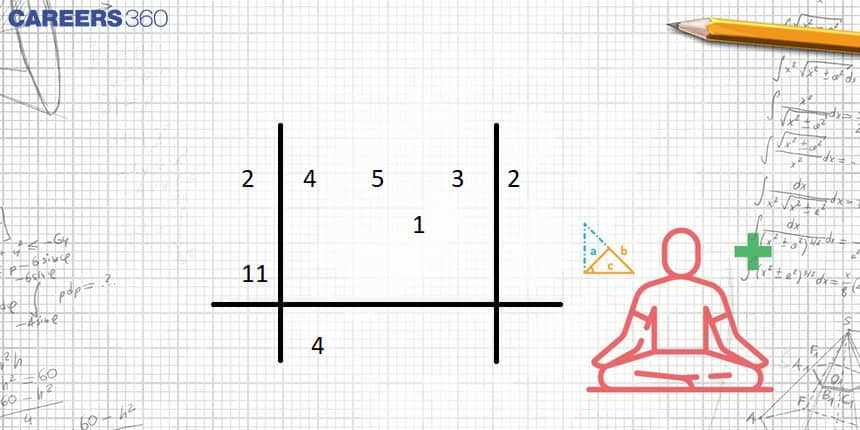###### Synopsis

Vedic mathematics offers efficient techniques for dividing large numbers. Techniques such as "Paravartya Yojayet" and "Nikhilam Navatashcaramam Dashatah" convert the traditional process of division into a faster and efficient one . Let’s understand the step-by-step process of dividing numbers using these.###### Synopsis

Vedic mathematics offers efficient techniques for dividing large numbers. Techniques such as "Paravartya Yojayet" and "Nikhilam Navatashcaramam Dashatah" convert the traditional process of division into a faster and efficient one . Let’s understand the step-by-step process of dividing numbers using these.

Vedic mathematics is a collection of mathematical techniques and methods that are said to be based on the teachings of ancient Indian sages. It is known for providing efficient and alternative methods of solving mathematical problems. Vedic mathematics can be applied in the field of division to speed up the process. Following are the three methods of Division used in Vedic Maths. The first two are useful in case the number is near to the base (power of 10) and the last is a general method.

### 1. Nikhilam Navatascaramam Dasatah

This method is particularly useful if the divisor is near the base or less than the base. Following is the structure for division:The Nikhilam sutra in Vedic mathematics modifies the divisor and places it in the leftmost position. The number of digits from the right side of the dividend that is equal to the number of zeros in the base is put in the remainder. The rest of the dividend is put in the middle, or quotient part. Instead of subtraction, this method uses addition, making it simpler than traditional division methods.

The algorithm for Nikhilam sutra is as follows:

1. The leftmost digit in the quotient part is considered the first digit of the quotient. This digit is multiplied by the modified divisor, and the result is added to the next digit.

2. The result of the addition is then multiplied by the modified divisor and added to the next digit.

3. This process is repeated until the last digit of the quotient part is reached. The results of the additions in this part will give the quotient. In this part, at each place, the rightmost digit (the unit digit) is placed as it is, and any extra digit is carried over to the immediate left part.

4. If the addition in the remainder part is greater than the divisor, it is divided again by the divisor, and the quotient obtained is added to the original quotient to get the final quotient. The last remainder is considered the final one of the process.

#### Example:

Dividend : 12,341

Divisor : 99

The base is 100, since 99 is closer to 100. Deviation is calculated by applying the sutra “Nikhilam Navatascaramam Dasatah”.

Modified Divisor (M.D.): 01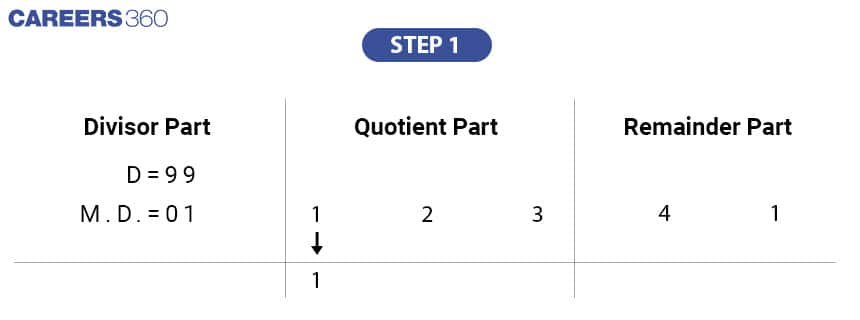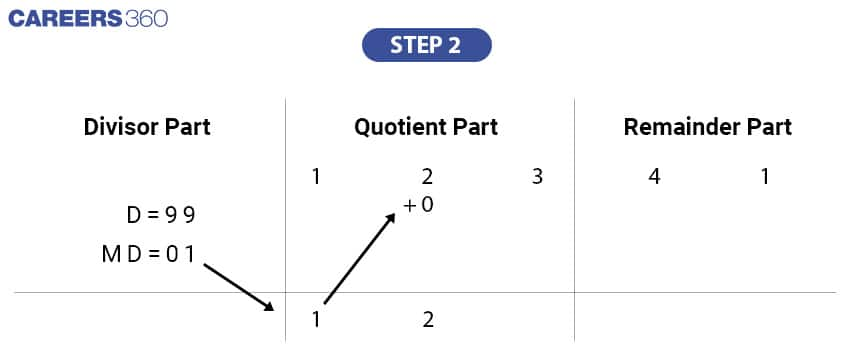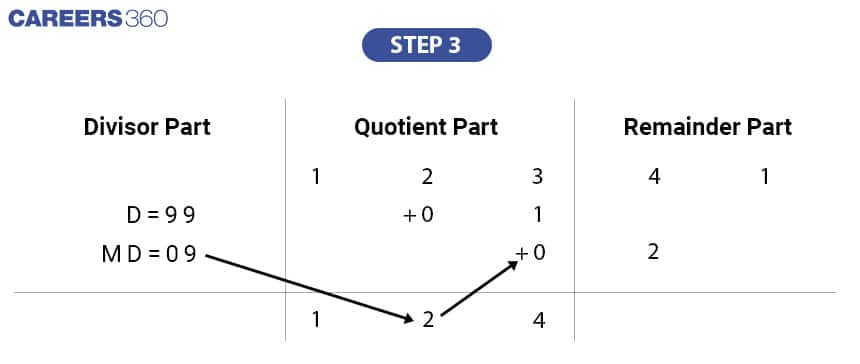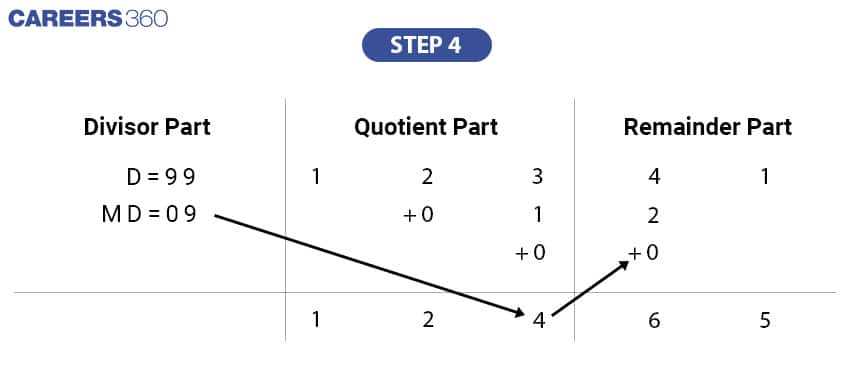2. Paravartya Yojayet

This method is used for long division when the divisor is close to the base and greater than the base. In this method, the difference between the divisor and the base is calculated, and the sign of each digit in this difference is changed to negative. This new number is called the "paravartya" of the divisor. The long division process is then performed using this Paravartya instead of the original divisor, following the same steps as in the Nikhilam method.

Let’s understand this using the help of an example.

#### Example

Dividend: 1,36,972

Divisor: 121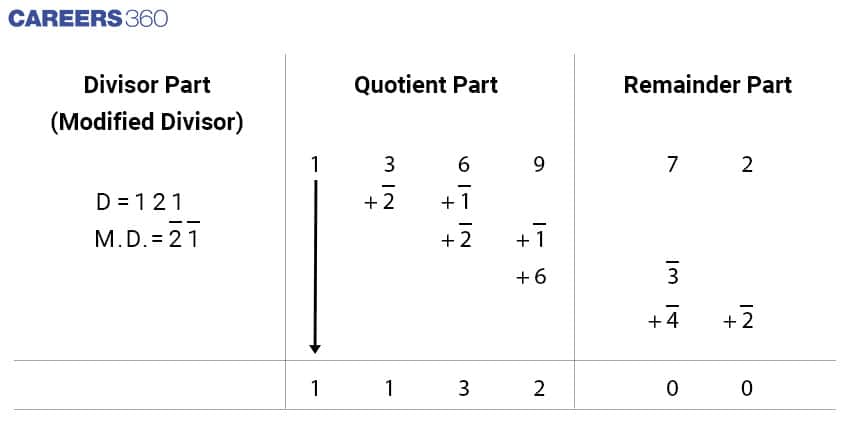3. Dhwajank (Flag Digit)

The "Dhwajank method" (also known as the "flag digit method") is a method of division where the divisor is split into two parts. The left part is called the "principal divisor" and the right part is referred to as the "Dhwajank" or "flag digit". The division is performed using only the principal divisor, while both the principal divisor and the Dhwajank are placed in the divisor section.

The algorithm for performing division using the Dhwajank (flag digit) method is as follows:

1. Split the dividend into two parts, with the number of digits in the Dhwajank determining the number of digits in the remainder. The remaining digits of the dividend make up the quotient.

2. During each step of the division, the principal divisor divides the true dividend and the remainder is recorded. This forms the gross dividend (GD).

3. In each step, the product of Urdhva Tiryagbhyam (vertically and crosswise) sutra is subtracted from the gross dividend to find the true dividend. The principal divisor is then used to divide the true dividend and find the next digit of the quotient and the remainder.

4. If at any point the Urdhva Tiryak product is greater than the gross dividend, the division process cannot proceed. In this case, the previous digit of the quotient is decreased by 1 in order to obtain the proper gross dividend for subtracting the Urdhva Tiryak product.

Let’s understand the algorithm using the help of an example:

Dividend: 23,754

Divisor: 74

Solution:

Step 1: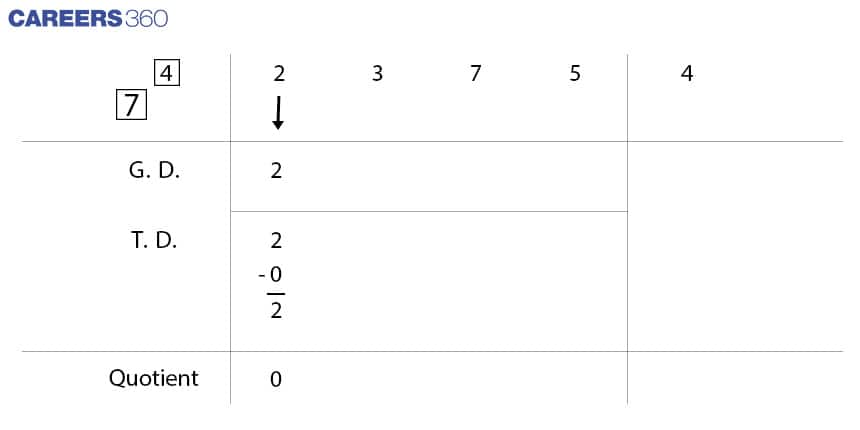Step 2: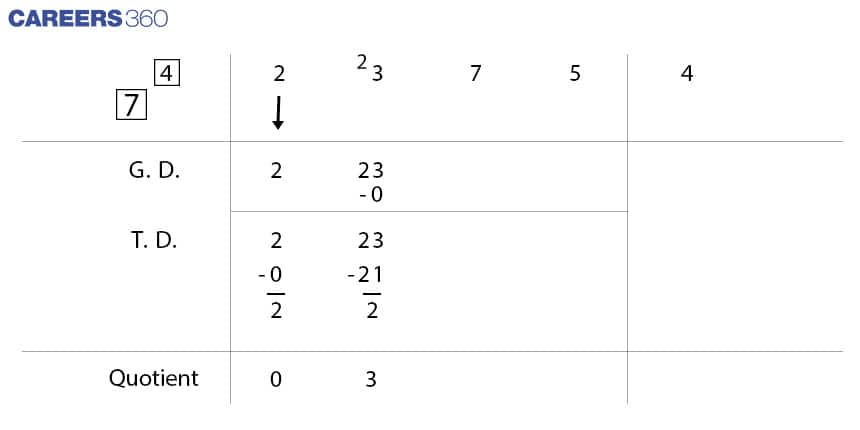Step 3: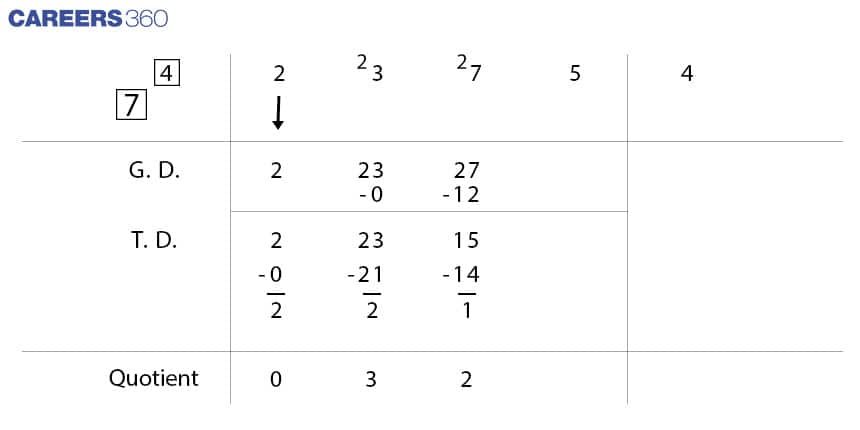Step 4: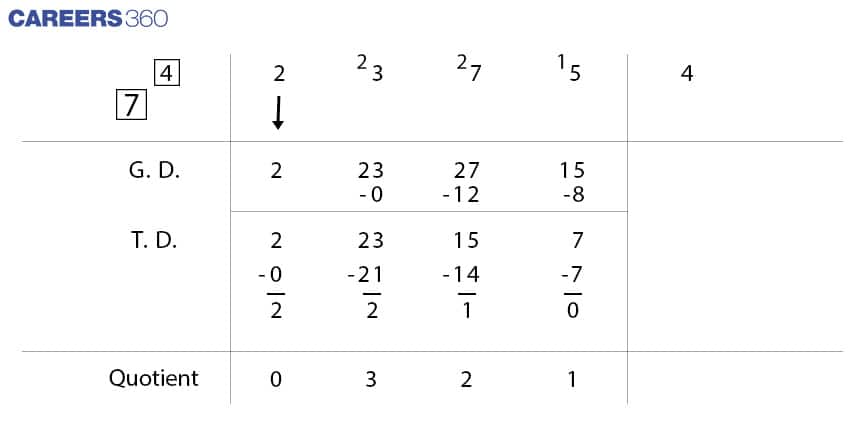Step 5: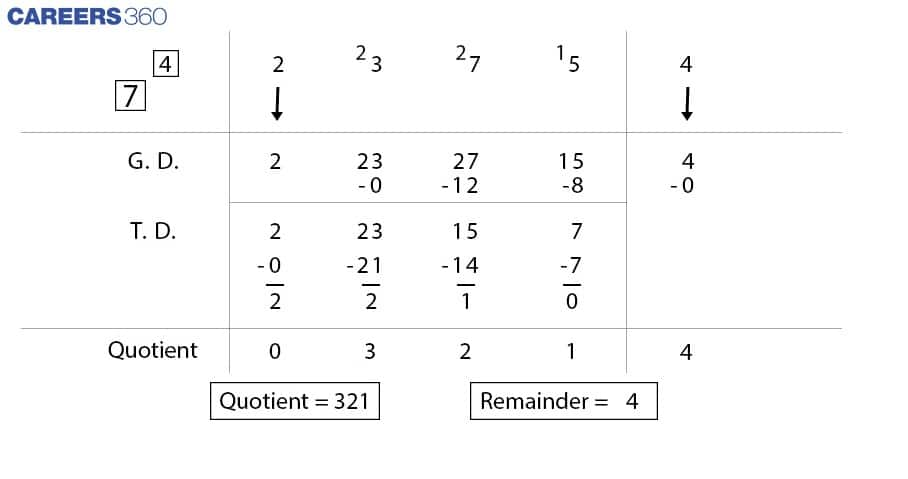It is possible to divide 23,754 by 74 using traditional mathematical methods on pen and paper. However, there are two points to be noted in this case: the first is obviously proficiency in multiplication and subtraction is required and secondly, without the use of a calculator, traditional methods of division are most efficient when the table of divisors are already memorised, which is unrealistic in case of large numbers. Vedic mathematics can provide an advantage in such scenarios, as it allows for the completion of such divisions on pen and paper in a relatively short amount of time and with less tedious calculations.

• Mathematics
• Applied mathematics
• Abstract algebra
• Arithmetic
• Elementary mathematics
• Operations on numbers
• Algorithms
• Elementary arithmetic
• Binary operations
• Theoretical computer science

## Careers360 helping shape your Career for a better tomorrow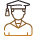#### 250M+

Students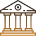#### 30,000+

Colleges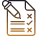#### 500+

Exams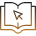E-Books